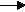gcsescience.com                                                 15                                                 gcsescience.com

Extraction of Metals

Aluminium - Anodising.

The thickness of the oxide layer on the surface of aluminium metal
can be increased by a process called anodising.
This gives the aluminium extra corrosion resistance.

The aluminium metal is treated with sodium hydroxide solution
which removes the thin oxide layer on the surface.
The clean aluminium metal is then used as the anode
for the electrolysis of dilute sulfuric acid.
Oxygen gas which is given off at the anode reacts with the
surface of the aluminium and forms a thick oxide layer.

The half equation at the anode is
2Al(s)  +  6OH-(aq)  -  6e-Al2O3(s)  +  3H2O(l)

Hydrogen gas is given off at the cathode.
The half equation at the cathode is
6H+(aq)   +  6e-3H2(g)

gcsescience.com      The Periodic Table      Index      Metal Quiz      gcsescience.com